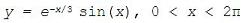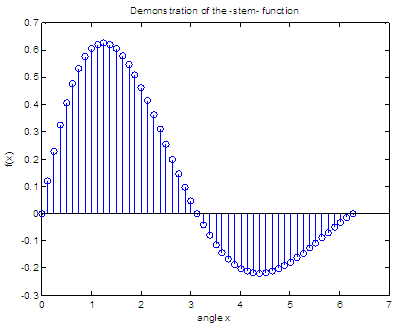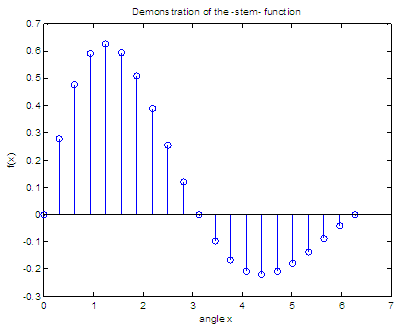# Matlab Plot - stem

In this example, we study the 'stem' instruction to plot Matlab functions.

It draws vertical lines (with a little circle at the tip) proportional to the value of the function at that particular horizontal value. 'stem' does not join the circles with a line, and it is very helpful to stress the fact that the function is not continuous but discrete.

Let's assume that we want to plot the following elegant exponential and sinusoidal function:### Example: let's draw a simple discrete function

We can develop a script like this:

% Avoid superimposed operations and close previous figs.
clc; clear; close all

% First, we define 51 values of our independent variable
x = 0 : 2*pi/50 : 2*pi;

% Second, we define the function to graph
y = exp(-x/3) .* sin(x);

% Third, we use the 'stem' function to plot discrete values
stem(x,y)

% We can add title and labels (as strings in arguments)
title('Demonstration of the -stem- function')
xlabel(
'angle x')
ylabel(
'f(x)')

And we get the following plot:If we define our independant variable using less points, as in

x = 0 : 2*pi/20 : 2*pi;

we get the following visual change:From 'Matlab Plot' to home

From 'Matlab Plot' to '2D Plot Menu'